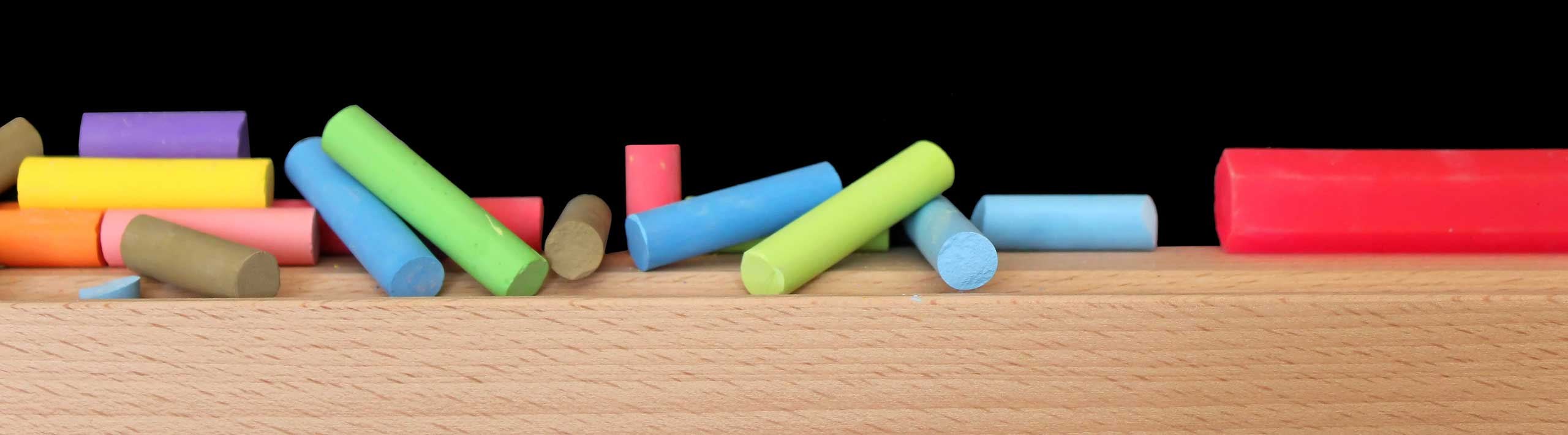# Rationalize Denominators Questions with Solutions

Grade 10 questions on how to rationalize radical expressions with solutions are presented.

## To rationalize radical expressions with denominators is to express the denominator without radicals

The following identities may be used to rationalize denominators of rational expressions.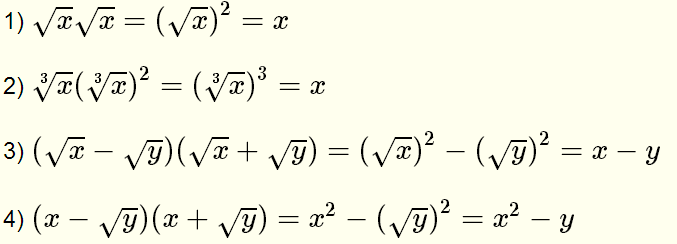### Examples

Rationalize the denominators of the following expressions and simplify if possible.### solution

Because of √2 in the denominator, multiply numerator and denominator by √2 and simplify### solution

Because of 3√x in the denominator, multiply numerator and denominator by (3√x)2 and simplify### solution

Because of the expression √3 - √2 in the denominator, multiply numerator and denominator by its conjugate √3 + √2 to obtain### solution

Because of the expression 3√(x2) in the denominator, multiply numerator and denominator by (3√(x2))2 to obtain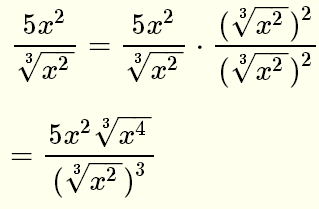Simplify and cancel terms### solution

Because of the expression y + √(x2+y2) in the denominator, multiply numerator and denominator by its conjugate y - √(x2 + y2) to obtainRationalize the denominators of the following expressions and simplify if possible.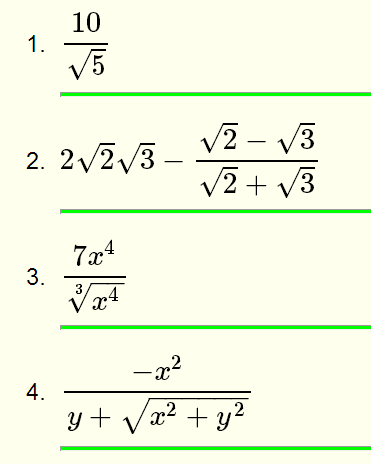### Solutions to the Above Problems

1. Multiply numerator and denominator by √5and simplify2. Multiply numerator and denominator by √2 - √3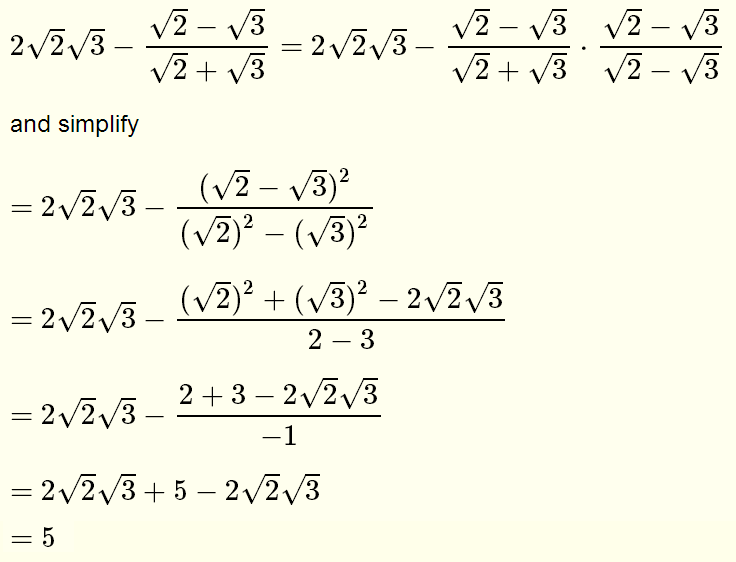3. Multiply numerator and denominator by (3√(x4))2and simplify4. Multiply numerator and denominator by y - √(x2 + y2)and simplify